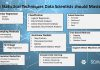## Here Are 10 Statistical Techniques Data Scientists Should Master### 1. Linear Regression

In statistics, linear regression is a linear approach to modeling the relationship between a scalar response (or dependent variable) and one or more explanatory variables (or independent variables). The case of one explanatory variable is called simple linear regression. For more than one explanatory variable, the process is called multiple linear regression. This term is distinct from multivariate linear regression, where multiple correlated dependent variables are predicted, rather than a single scalar variable.

### 2. Classification

In machine learning and statistics, classification is the problem of identifying to which of a set of categories (sub-populations) a new observation belongs, on the basis of a training set of data containing observations (or instances) whose category membership is known. Examples are assigning a given email to the “spam” or “non-spam” class, and assigning a diagnosis to a given patient based on observed characteristics of the patient (sex, blood pressure, presence or absence of certain symptoms, etc.). Classification is an example of pattern recognition.

### 3. Resampling

In statistics, resampling is any of a variety of methods for doing one of the following:

• Estimating the precision of sample statistics (medians, variances, percentiles) by using subsets of available data (jackknifing) or drawing randomly with replacement from a set of data points (bootstrapping)
• Exchanging labels on data points when performing significance tests (permutation tests, also called exact tests, randomization tests, or re-randomization tests)
• Validating models by using random subsets (bootstrapping, cross-validation)

### 4. Shrinkage

In statistics, shrinkage has two meanings:

• In relation to the general observation that, in regression analysis, a fitted relationship appears to perform less well on a new data set than on the data set used for fitting. In particular the value of the coefficient of determination ‘shrinks’. This idea is complementary to overfitting and, separately, to the standard adjustment made in the coefficient of determination to compensate for the subjunctive effects of further sampling, like controlling for the potential of new explanatory terms improving the model by chance: that is, the adjustment formula itself provides “shrinkage.” But the adjustment formula yields an artificial shrinkage, in contrast to the first definition.
• To describe general types of estimators, or the effects of some types of estimation, whereby a naive or raw estimate is improved by combining it with other information (see shrinkage estimator). The term relates to the notion that the improved estimate is at a reduced distance from the value supplied by the ‘other information’ than is the raw estimate. In this sense, shrinkage is used to regularize ill-posed inference problems.

### 5. Dimension Reduction

In statistics, machine learning, and information theory, dimensionality reduction or dimension reduction is the process of reducing the number of random variables under consideration by obtaining a set of principal variables. It can be divided into feature selection and feature extraction.

### 6. Nonlinear Models

The rapid and sustained increases in computing power starting from the second half of the 20th century have had a substantial impact on the practice of statistical science. Early statistical models were almost always from the class of linear models, but powerful computers, coupled with suitable numerical algorithms, caused an increased interest in nonlinear models (such as neural networks) as well as the creation of new types, such as generalized linear models and multilevel models.

Read the source article in AISoma.de.